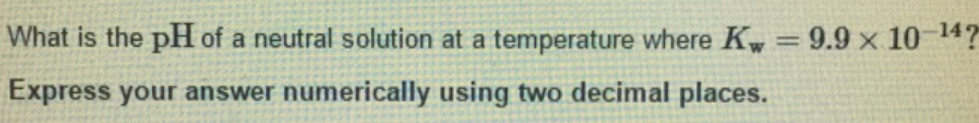# Problem: What is the pH of a neutral solution at a temperature where Kw = 9.9 times 10-14? Express your answer numerically using two decimal places.

###### FREE Expert Solution
100% (37 ratings)###### Problem Details

What is the pH of a neutral solution at a temperature where Kw = 9.9 times 10-14? Express your answer numerically using two decimal places.